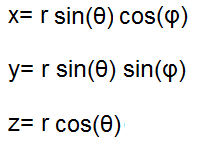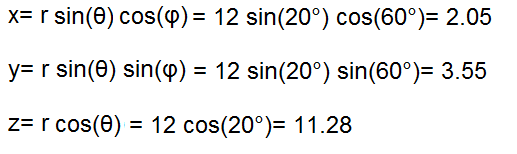﻿ Spherical to Cartesian Coordinates Calculator ﻿# Spherical to Cartesian Coordinates Calculator### Spherical to Cartesian (Rectangular) Coordinate System DiagramThis cartesian (rectangular) coordinates converter/calculator converts the spherical coordinates of a unit to its equivalent value in cartesian (rectangular) coordinates, according to the formulas shown above.

Spherical coordinates are depicted by 3 values, (r, θ, φ). When converted into cartesian coordinates, the new values will be depicted as (x, y, z).

To use this calculator, a user just enters in the (r, θ, φ) values of the spherical coordinates and then clicks 'Calculate', and the cartesian coordinates will be automatically computed and shown below.

By default, the calculator will compute the result in degrees. However, by using the drop-down menu, the option can changed to radians, so that the result can also be computed in radians.

Example Calculation

Convert the spherical coordinates (12, 20°, 60°) into its equivalent cartesian coordinate.This answer is calculated in degrees mode.

Related Resources

﻿